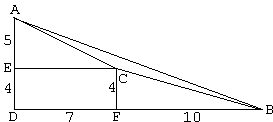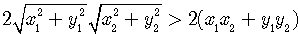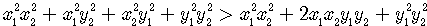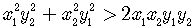# Sam Loyd's Geometric Puzzle

I've been thumbing through Mathematical Puzzles of Sam Loyd selected and edited by Martin Gardner (Dover, 1959), when I came across a puzzle whose set up and the original solution by Sam Loyd amused me greatly. This is the puzzle #36 from the book.The puzzle is framed into a story of an auction at which a plot of land comprising three squares surrounding a triangular lake was offered for sale. Sam Loyd states the puzzle thus:

The question I ask our puzzlists is to determine how many acres there would be in that triangular lake, surrounded as shown by square plots of 370, 116 and 74 acres. The problem is of peculiar interest to those of a mathematical turn, in that it gives a positive and definite answer to a proposition which, according to usual methods, produces one of those ever-decreasing, but never-ending decimal fractions.

More than by the puzzle itself I was amused by Sam Loyd's ingenious solution.### Other Puzzles by Sam Loyd### Sam Loyd's SolutionForm a right-angled triangle ABD with sides 9 = 4 + 5 and 17 = 7 + 10. By the Pythagorean theorem, the square of the hypothenuse AB is given by AB2 = 92 + 172 = 81 + 289 = 370 - exactly the size in acres of the biggest square in the diagram. Thinking of D as the origin, find point C with coordinates (7, 4). Let CE ⊥ AD, and CFBD.

In ΔACE, the Pythagorean theorem gives

AC2 = 52 + 72 = 25 + 49 = 74

- another square in the diagram. To get the third square apply the Pythagorean theorem to ΔBFC:

BC2 = 42 + 102 = 16 + 100 = 116,

which proves that ΔABC is exactly of the shape of the triangular lake. Now then

 Area(ABC) = Area(ABD) - Area(ACE) - Area(BFC) - Area(CEDF) = 9·17/2 - 5·7/2 - 4·10/2 - 4·7.

We thus obtain Area(ABC) = 76.5 - 17.5 - 20 - 28 = 11. As Sam Loyd notes:

In this remarkable problem we find that the lake contains exactly 11 acres, therefore the approximate answer of "nearly 11 acres" is not sufficiently correct.

So, what's interesting about this solution? The problem of finding area of a triangle given its three sides is routinely solved with Heron's formula. I can't imagine that Sam Loyd was unaware of this route. More likely he counted on general ignorance of the formula even among mathematics fans and puzzlists. With Heron's formula, the solution is straightforward and requires just a few algebraic steps based on repeated application of the well-known formula:

(X - Y)(X + Y) = X2 - Y2

Sides of ΔABC are defined by a2 = 116, b2 = 74, and c2 = 370. From Heron's formula

16·Area(ABC)2 = (a + b + c)(a + b - c)(c + b - a)(c - b + a)

In other words,

 16·Area(ABC)2 = ((a + b)2 - c2)(c2 - (a - b)2) = (2ab + (a2 + b2 - c2))(2ab - (a2 + b2 - c2)) = 4a2b2 - (a2 + b2 - c2)2,

which, in our case, reduces to

16·Area(ABC)2 = 4·74·116 - (74 + 116 - 370)2 = 1936,

wherefrom Area(ABC)2 = 1936/16 = 121 = 112. This derivation suggests a nice little theorem: if the squares of the sides of a triangle are whole integers, then the square of its area is either a whole integer, or a half of a whole integer, or a quarter of a whole integer. No more may be claimed in general: the area itself may, of course, be irrational. Herein may lie an explanation to the approach taken by Sam Loyd. Loyd was generously giving away the way in which he arrived at a particular triple 74, 116, 370. Let integers x1, x2, y1, y2 be such that the point C on the diagram below is inside (as in Sam Loyd's puzzle) ΔABD. Having point C outside ΔABD changes computations very little, but changes them nonetheless, and Loyd should have verified this condition.Then all areas involved (ABD, ABC, ACE, BFC, CEDF) are either integers or half integers. (Pick's Theorem also comes to mind.) Area(ABC) can be found directly

 Area(ABC) = Area(ABD) - Area(ACE) - Area(BFC) - Area(CEDF) = (x1 + x2)(y1 + y2)/2 - x1y1/2 - x2y2/2 - x1y2 = (x2y1 - x1y2)/2

or by more general methods. Try it! The calculations are much simpler than offered by Sam Loyd. For the Area(ABC) to be integer, the expression x2y1 - x1y2 must be even. (With C outside ΔABD, we'd have to consider |x2y1 - x1y2|.) Thus we are getting a way to generate all such triples of squares that lead to an integer "lake" area.Martin Gardner (The Second Scientific American Book of Mathematical Puzzles and Diversions, The U. of Chicago Press, 1987, p. 32) mentions that in the 1890s Loyd collaborated with H.E. Dudeney on a series of puzzle articles for the English magazine Tit-Bits. Later they arranged to exchange puzzles for their magazines and newspaper columns. However it may be, but the above puzzle found its way into Dudeney's collection Amusements in Mathematics (Dover, 1970, #189):

I was on a visit to one of the large towns of Yorkshire. While walking to the railway station on the day of my departure a man thrust a handbill upon me, and I took this into the railway carriage and read it at my leisure. It informed me that three Yorkshire neighbouring estates were to be offered for sale. Each estate was square in shape, and they joined one another at their corners, just as shown in the diagram. Estate A contains exactly 370 acres, B contains 116 acres, and C 74 acres.

Now, the little triangular bit of land enclosed by the three square estates was not offered for sale, and, for no reason in particular, I became curious as to the area of that piece. How many acres did it contain?

I find it remarkable that the verbiage of the problem has been modified, but the numeric data was not. The solutions are virtually identical as well. How easy it is to modify the numeric data? Let's, for example, take x1 = 5, x2 = 7, y1 = 5, and y2 = 3. This would give us three squares: 50 = 52 + 52, 58 = 32 + 72, and 208 = (3+5)2 + (5+7)2, with the triangle area equal to

(3 + 5)(5 + 7)/2 - 5·5/2 - 3·7/2 - 3·5 = 10,

which could be also obtained simply as (7·5 - 3·5)/2. Is it that simple? Well, there is one point that has been glossed over. We should have verified that a triangle indeed exists whose sides have squares 50, 58, 208. That is to say, we had to check that the square roots of numbers 50, 58, and 208 satisfy three triangle inequalities. While two are obvious, the third one 50 + 58 > 208 is less so. Will you please check it?

Now, more generally, let four numbers x1, x2, y1, and y2 be chosen in such a way that x2y1 - x1y2 ≠ 0. Does it follow from here that three segments formed according to Loyd/Dudeney's solution form a triangle? The answer is Yes, and you are welcome to verify it. The question as to why Loyd and Dudeney used different story setups but exactly the same numeric data is more difficult to answer. You are also welcome to ponder this point over.We want to verify thatSince all terms are positive squaring leads to an equivalent inequality which, after some simplifications, appears to beSquaring once more we getwhich simplifies toand finally toWhich is equivalent to x2y1 - x1y2 ≠ 0.